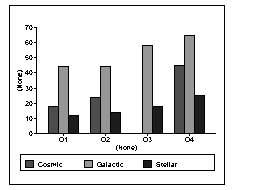## Populating a graph with data

This section shows how you can populate an empty graph with data.

`graphName.AddSeries ( seriesName )`

AddSeries returns an integer that identifies the series that was created. The first series is numbered 1, the second is 2, and so on. Typically you use this number as the first argument in other graph functions that manipulate the series.

So to create a series named Stellar, code:

```int SNum

`graphName.AddData ( seriesNumber, value, categoryLabel )`

The first argument to AddData is the number assigned by PowerBuilder to the series. So to add two data points to the Stellar series, whose number is stored by the variable SNum (as shown above), code:

```gr_1.AddData(SNum, 12, "Q1") // Category is Q1
gr_1.AddData(SNum, 14, "Q2") // Category is Q2```

### Getting a series number

You can use the FindSeries function to determine the number PowerBuilder has assigned to a series. FindSeries returns the series number. This is useful when you write general-purpose functions to manipulate graphs.

An example

Say you want to graph quarterly printer sales. Here is a script that populates the graph with data:

```gr_1.Reset( All! ) // Resets the graph.
// Create first series and populate with data.

int SNum
gr_1.AddData(SNum, 12, "Q1") // Category is Q1.
gr_1.AddData(SNum, 14, "Q2") // Category is Q2.
gr_1.Adddata(SNum, 18, "Q3") // Category is Q3.
gr_1.AddData(SNum, 25, "Q4") // Category is Q4.
// Create second series and populate with data.
// Use the same categories as for series 1 so the data
// appears next to the series 1 data.
// Create third series and populate with data.